# Area expansion is like a photographic enlargement

 Every linear dimension increases by the same percentage with a change in temperature, including holes. This assumes that the expanding material is uniform. .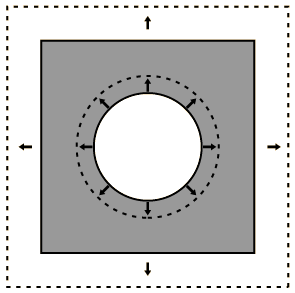Thermal expansion of an area can be calculated from the linear expansion coefficient.
 Table of expansion coefficients
Index

Expansion concepts
HyperPhysics***** Thermodynamics Go Back

# Area Expansion

Over small temperature ranges, the thermal expansion is described by the coefficient of linear expansion. If the linear expansion is put in the form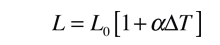then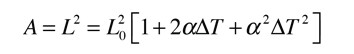In most cases the quadratic term above can be neglected since the typical expansion coefficient is on the order of parts per million per degree C. The expression then becomes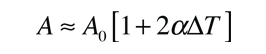which is equivalent to the expression at left.
 Will a hole in a uniform metal plate get larger or smaller with heating?
Index

Expansion concepts
HyperPhysics***** Thermodynamics Go Back

# Volume Expansion

 Over small temperature ranges, the thermal expansion is described by the coefficient of linear expansion. If the linear expansion is put in the formthen the expanded volume has the form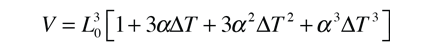In most cases the quadratic and cubic terms above can be neglected since the typical expansion coefficient is on the order of parts per million per degree C. The expression then becomes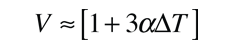Index

Expansion concepts
HyperPhysics***** Thermodynamics Go Back